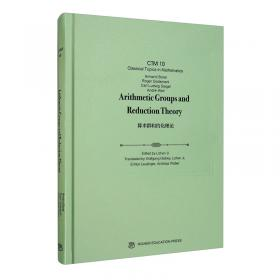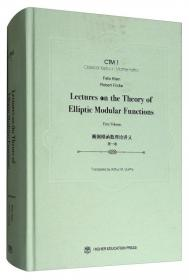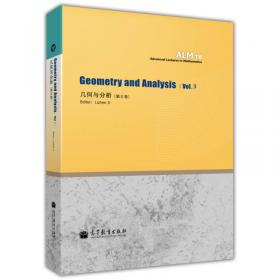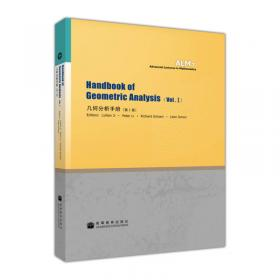# 群的上同调与代数K-理论2009-09

ISBN: 9787040266269

• 　　Cohomology of groups is a fundamental tool in many subjects in modernmathematics. One important generalized cohmnology theory is the algebraic Ktheory，and algebraic K-groups of rings such as rings of integers and group ringsare important invariants of the rings. They have played important roles in algebra，geometric and algebraic topology， number theory， representation theory etc. Cohomologyof groups and algebraic K-groups are also closely related. For example，algebraic K-groups of rings of integers in number fields can be effectively studiedby using cohomology of arithmetic groups. Arthur Bartels and Wolfgang Liick: On Crossed Product Rings with Twisted Involutions, Their Module Categories and L-Theory

Oliver Baues: Deformation Spaces for A~ne Crystallographic Groups...

Kenneth S. Brown : Lectures on the Cohomology of Groups

Daniel R. Grayson : A Brief Introduction to Algebraic K-Theory

Daniel duan-Pineda and Silvia Millan-Lopez : The Braid Groups of RP2 Satisfy the Fibered Isomorphism Conjecture

Max Karoubi: K-Theory, an Elementary Introduction

Max Karoubi: Lectures on K-Theory.

Wolfqang Liick: On the Farrell-Jones and Related Conjectures

Stratos Prassidis: Introduction to Controlled Topology and Its Applications

tIouron9 Qin: Lecture Notes on K-Theory

Daniel Quillen: Higher Algebraic K-Theory: I

Daniel Quillen : Finite Generation of the Groups K, of Rings of Algebraic Integers

David Rosenthal: A User's Guide to Continuously Controlled Algebra

Christophe Sould(Notes by Marco Varisco): Higher K-Theory of Algebraic Integers and the Cohomology of Arithmetic Groups
• ##### 内容简介:
Cohomology of groups is a fundamental tool in many subjects in modernmathematics. One important generalized cohmnology theory is the algebraic Ktheory，and algebraic K-groups of rings such as rings of integers and group ringsare important invariants of the rings. They have played important roles in algebra，geometric and algebraic topology， number theory， representation theory etc. Cohomologyof groups and algebraic K-groups are also closely related. For example，algebraic K-groups of rings of integers in number fields can be effectively studiedby using cohomology of arithmetic groups.
• ##### 目录:
Arthur Bartels and Wolfgang Liick: On Crossed Product Rings with Twisted Involutions, Their Module Categories and L-Theory

Oliver Baues: Deformation Spaces for A~ne Crystallographic Groups...

Kenneth S. Brown : Lectures on the Cohomology of Groups

Daniel R. Grayson : A Brief Introduction to Algebraic K-Theory

Daniel duan-Pineda and Silvia Millan-Lopez : The Braid Groups of RP2 Satisfy the Fibered Isomorphism Conjecture

Max Karoubi: K-Theory, an Elementary Introduction

Max Karoubi: Lectures on K-Theory.

Wolfqang Liick: On the Farrell-Jones and Related Conjectures

Stratos Prassidis: Introduction to Controlled Topology and Its Applications

tIouron9 Qin: Lecture Notes on K-Theory

Daniel Quillen: Higher Algebraic K-Theory: I

Daniel Quillen : Finite Generation of the Groups K, of Rings of Algebraic Integers

David Rosenthal: A User's Guide to Continuously Controlled Algebra

Christophe Sould(Notes by Marco Varisco): Higher K-Theory of Algebraic Integers and the Cohomology of Arithmetic GroupsHandbook of Group Actions（群作用手册）（第V卷）Handbook of Group Actions（群作用手册）（第III 卷）Hodge理论和L2分析（英文版）